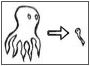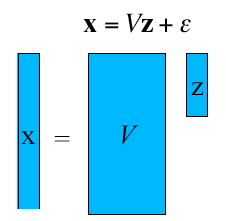ModelReduction.rudnyi.ru

 Home Model Reduction Applications Software
 MOR Home Linear Second Order Parametric Weakly Nonlinear Nonlinear

# Model Order Reduction

Model reduction or model order reduction is a mathematical theory to find a low-dimensional approximation for a system of ordinary differential equations (ODEs). The main idea is that a high-dimensional state vector is actually belongs to a low-dimensional subspace as shown in Fig. 1.Fig. 1. Idea of a low-dimensional subspace.

Provided that the low-dimensional subspace is known, the ordinary differential equations can be probjected on it. This is showin in Fig. 2 in the case of a linear system of ODEs. The projection gives us a required low-dimensional approximation.Fig. 2. Projection a system of ODEs on to a low-dimensional subspace.

The goal of the mathematical theory is to find such a low-dimensional subspace. Available methods for model reduction are classified as follows.

Page Linear describes model reduction for a linear system of ODEs of the first order.

Page Second Order describes model reduction for a linear system of ODEs of the second order.

Page Parametric describes model reduction for a linear system of ODEs when it is necessary to preserve some parameters within system matrices.

Page Weakly Nonlinear describes model reduction for a nonlinear system of ODEs but when nonlinearity is limited to quadratic and cubic terms.

Page Nonlinear describes model reduction for a general nonlinear system of ODEs.

Note that model reduction is an active area of research and not everything is presented at this site.

## Books, reviews

Dimension Reduction of Large-Scale Systems.
Benner, P., Mehrmann, V., Sorensen, D. (eds).
Lecture Notes in Computational Science and Engineering (LNCSE). Springer-Verlag, Berlin/Heidelberg, Germany, v. 45, 2005,
Book at Springer.

Special Issue on Order Reduction of Large-Scale Systems.
Peter Benner, Roland W. Freund, Danny C. Sorensen and Andras Varga (eds).
Linear Algebra and its Applications Volume 415, Issues 2-3, Pages 231-578 (1 June 2006)
Issue at ScienceDirect.

Evgenii B. Rudnyi

Designed by
Masha Rudnaya